|

# 耐热珍稀红木 大卫23℃纯实木地热地板评测

人体正常体温37℃乘以黄金比例0.618约为23℃，这个温度是人体感觉最舒服的，所以，23℃是人类居住永恒的追求。世界上适宜人居住的城市都会有一个共同点，温差较小，气候宜人，空气清新。23℃，不仅仅是一个温度，更是地板制造艺术的高度。

大卫23℃纯实木地热地板，将岁月珍稀原木与高品质居住生活完美融合。探索岁月珍稀原木的奥秘，挖掘木材天然的肌理，采用大卫独有微波活性烘干技术保持稳定性，不易起翘、不易变形；高分子UV环保油漆，宜居自然健康，热传导更快，足下升温，自下而上，调节温度，使整体居室温度均匀，即使老人、婴儿、孕妇，也可完全放心使用。

追寻人类居住的终极需求，今天搜狐焦点家居将评测大卫23℃纯实木地热地板——阔叶黄檀，看这款地板是否可以满足人们对于生活品质的孜孜追求。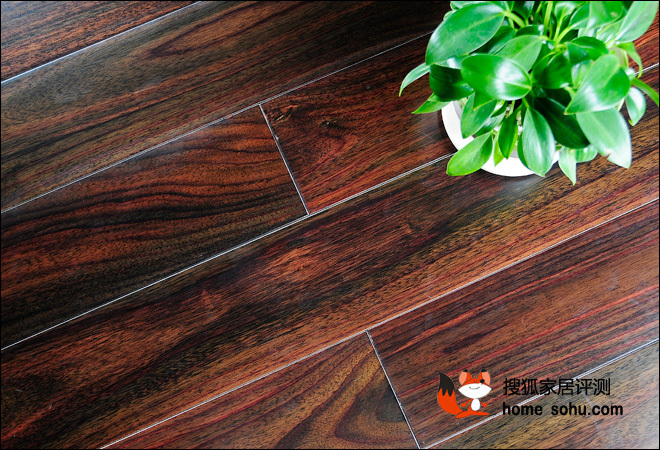大卫23℃纯实木地热地板评测

大卫23℃纯实木地热地板基本信息

评测品牌：大卫地板

评测产品：23℃纯实木地热地板

产品规格：910×115×18（mm）

产品树种：阔叶黄檀（黑酸枝）

木材来源：东南亚

参考价格：3986元/㎡

评测时间：2014年7月17日

评测地点：搜狐焦点家居华东评测中心

【大卫23℃纯实木地热地板外观及细节评测】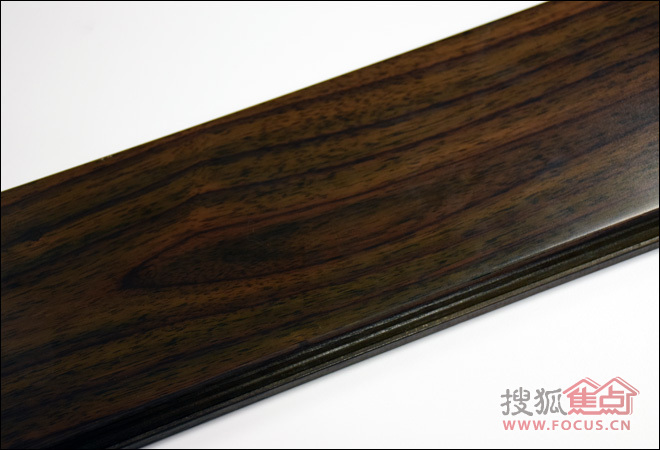卫23℃纯实木地热地板纹理

大卫23℃纯实木地热地板采用阔叶黄檀打造，纹理交错，色泽饱满，紫黑色条纹较宽，像是木屑酒精浸出液的紫色调，犹如一朵美丽的印度玫瑰，所以这种木材又名印度玫瑰木，是真正的红木。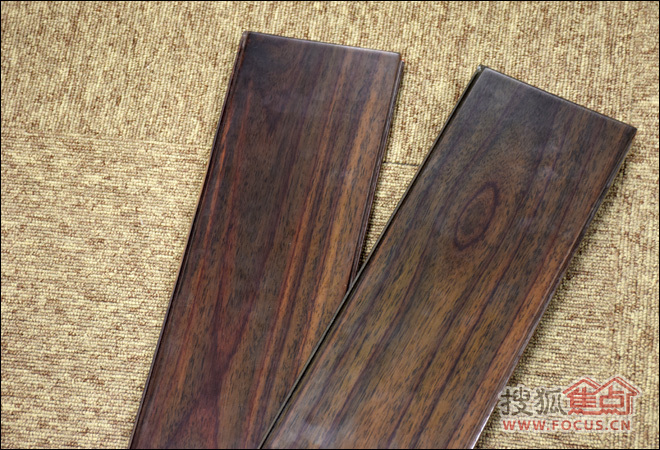大卫23℃纯实木地热地板外观

地板表面深深浅浅的木材天然纹理，具有意想不到的层次感，手触摸表面比较细腻，可闻到淡淡的木材香气，其中还夹杂着一丝酸酸的味道，也就是木材本身的味道，所以此木材又名黑酸枝，是国家红木标准中规定的红木品种。将大卫地板翻转过来，地板背面刻印着清晰的“大卫地板”的品牌logo，可见品牌细节之处的精致处理，非常到位。除此之外，地板背面木材的纹理依稀可见，深浅相间，常温下此种木材由内而外颜色会变深，阳光照射下会变浅。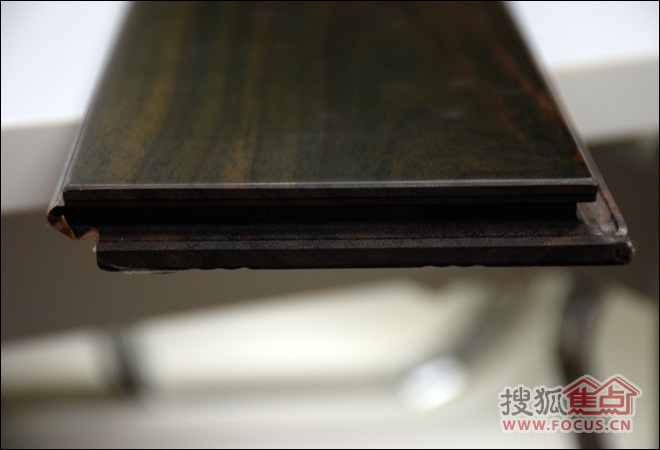大卫23℃纯实木地热地板锁扣

大卫此款实木地板的锁扣，经过特别设计后锁扣更大，双层保证，一扣即合，将两片木板牢牢扣住，更紧密，不拔缝、不起翘，稳定性更好，比较适应地热环境。此外，木材本身具有很强的耐热性。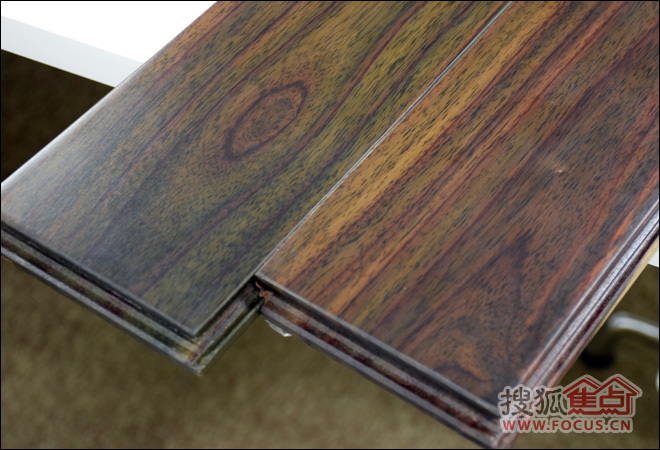大卫23℃纯实木地热地板拼接

锁扣拼接安装比较方便，两块地板拼接后，肉眼观察比较紧密，几乎没有缝隙，平时灰尘不容易藏匿于此，打理起来比较方便。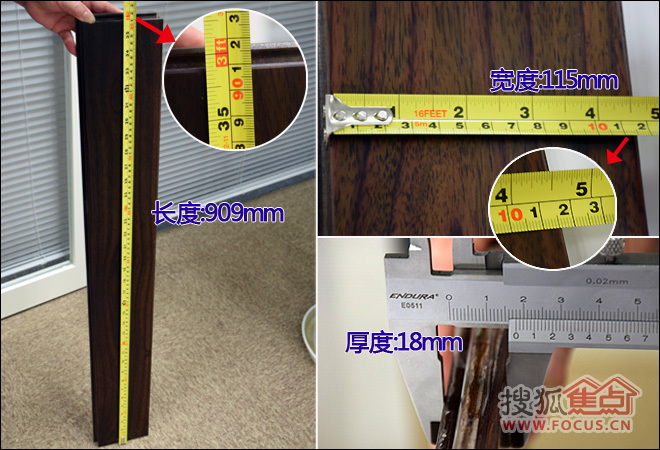大卫23℃纯实木地热地板规格

=====================================================

2013年大卫木业再次实现质的飞跃，全面研发生产木门、

http://www.daviefloor.com/

===========================================

阅读索引：

Page1： 大卫23℃纯实木地热地板外观及细节评测

Page2：大卫23℃纯实木地热地板功能性评测及总结

相关阅读：

·是实木？ 必美宝地雅爱新特复合木地板评测

·断背山专属地板 圣象V5系列阿兰达曲柳评测

耐热珍稀红木 大卫23℃纯实木地热地板评测 X

【大卫23℃纯实木地热地板功能性评测】

很多时候我们挑选地板，主要会考虑两点：一是要看材质是否具有很好的环保性，二是要看地板的具体性能，譬如平时好不好打理？防滑、耐磨度怎么样？水、防潮性能如何？

大卫这款阔叶黄檀纯实木地板，材质稀缺珍贵，外观高雅贵气，相信很多人都会被木材本身的香气吸引，但是作为要装饰到地面的地板，其性能还是比较受关注的。今天，搜狐焦点家居将对这款地板的基本性能：抗污、耐磨、防水、防滑和承重进行评测。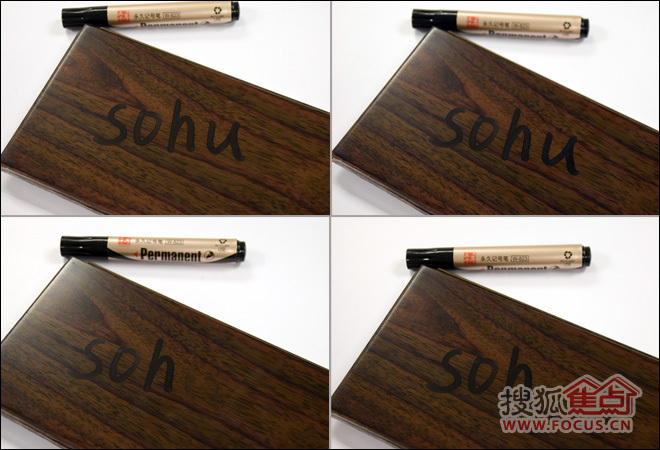大卫23℃纯实木地热地板抗污力测试

小编使用比较难清除的油性记号笔，在地板表面涂写。待其干透后，纸巾干擦几乎没可能去除字迹，沾取少量酒精涂在字迹上，轻轻擦拭便可去掉大部分字迹，少许用力几乎可以全部去除字迹。由此可见，这款地板抗污力还可以。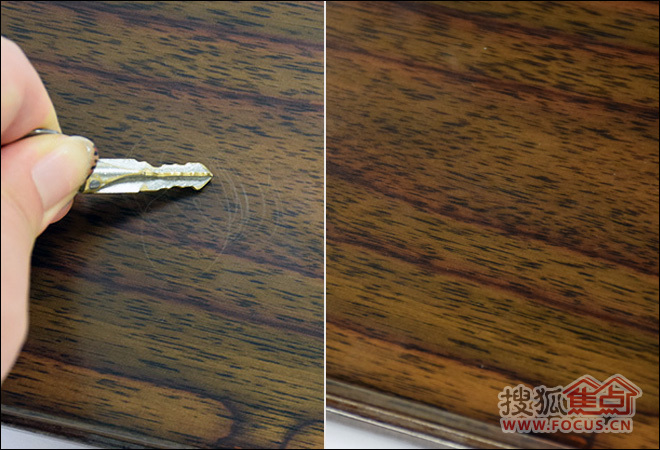大卫23℃纯实木地热地板耐磨测试

小编取出较尖锐的钥匙在地板表面不停划擦，30秒之后地板表面出现了明显划痕和少许碎屑，纸巾擦拭后基本可以恢复原样。所以，平时要注意对地板的打理，定期打蜡保养。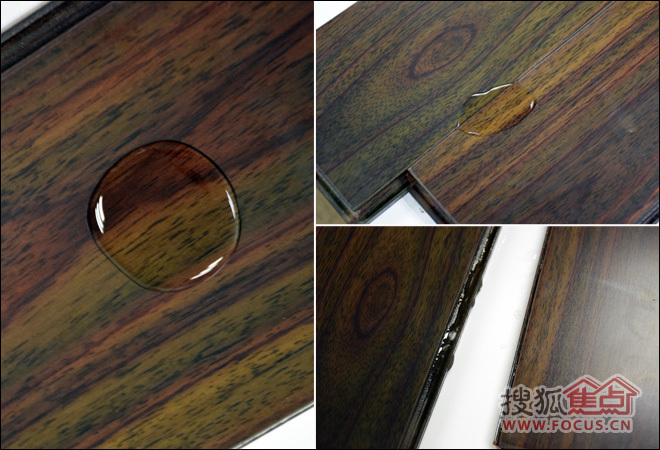大卫23℃纯实木地热地板防水测试

分别在地板表面和拼接处滴一滴清水，静置几分钟后，地板表面的水滴几乎没任何变化，也未渗透，地板拼接处的水滴出现了少量渗透。可见，此款地板表面防水性还可以，拼接严密度有待加强。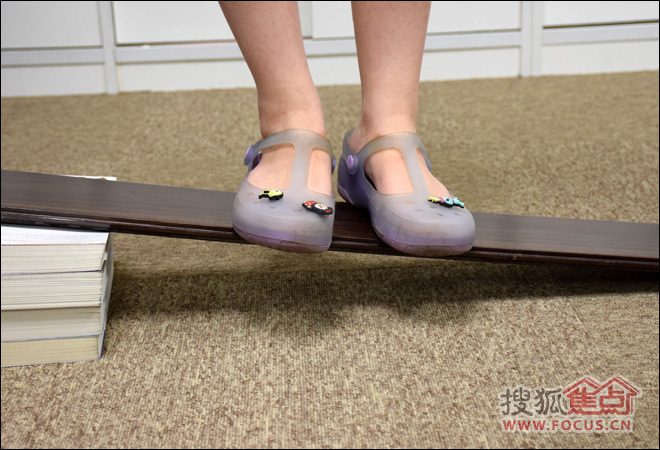大卫23℃纯实木地热地板承重测试

将地板一角垫起一定的角度，小编邀请一位同事双脚站上去大约1分钟的样子，地板没有断裂，但是稍微有些弯曲，可见此款实木地板承重能力还可以，还具有一定的韧性。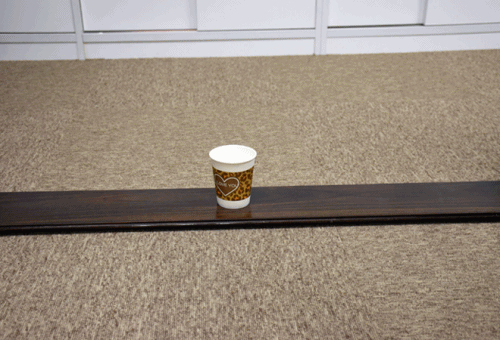大卫23℃纯实木地热地板防滑测试

放一杯装好水的纸杯放在地板表面，将地板依次抬起不同的角度，最高至大约30度时，纸杯才有滑落的迹象，可见此款地板的防滑性能不错。

【评测总结】

大卫23℃纯实木地热地板，抗污、防滑方面表现不错，但耐磨、防水方面稍逊一些。此款地板选用耐热珍稀原木——阔叶黄檀为原材，纹理清晰且独具韵味，表面采用高分子UV环保油漆，环保健康。使用微波活性烘干工艺，保留实木地板的天然实木纤维，稳定不变形，大锁扣设计牢靠更紧密，让这款地板适用于地热环境。由于取材于名贵树种精品红木，价格自然也是相对较高的，比较适合追求极致生活品质的人。

===========================================

阅读索引：

Page1： 大卫23℃纯实木地热地板外观及细节评测

Page2：大卫23℃纯实木地热地板功能性评测及总结

相关阅读：

·是实木？ 必美宝地雅爱新特复合木地板评测

·断背山专属地板 圣象V5系列阿兰达曲柳评测

`声明：本文由入驻焦点开放平台的作者撰写，除焦点官方账号外，观点仅代表作者本人，不代表焦点立场错误信息举报电话： 400-099-0099，邮箱：jubao@vip.sohu.com，或点此进行意见反馈，或点此进行举报投诉。`A B C D E F G H J K L M N P Q R S T W X Y Z
A - B - C - D - E
• A
• 鞍山
• 安庆
• 安阳
• 安顺
• 安康
• 澳门
• B
• 北京
• 保定
• 包头
• 巴彦淖尔
• 本溪
• 蚌埠
• 亳州
• 滨州
• 北海
• 百色
• 巴中
• 毕节
• 保山
• 宝鸡
• 白银
• 巴州
• C
• 承德
• 沧州
• 长治
• 赤峰
• 朝阳
• 长春
• 常州
• 滁州
• 池州
• 长沙
• 常德
• 郴州
• 潮州
• 崇左
• 重庆
• 成都
• 楚雄
• 昌都
• 慈溪
• 常熟
• D
• 大同
• 大连
• 丹东
• 大庆
• 东营
• 德州
• 东莞
• 德阳
• 达州
• 大理
• 德宏
• 定西
• 儋州
• 东平
• E
• 鄂尔多斯
• 鄂州
• 恩施
F - G - H - I - J
• F
• 抚顺
• 阜新
• 阜阳
• 福州
• 抚州
• 佛山
• 防城港
• G
• 赣州
• 广州
• 桂林
• 贵港
• 广元
• 广安
• 贵阳
• 固原
• H
• 邯郸
• 衡水
• 呼和浩特
• 呼伦贝尔
• 葫芦岛
• 哈尔滨
• 黑河
• 淮安
• 杭州
• 湖州
• 合肥
• 淮南
• 淮北
• 黄山
• 菏泽
• 鹤壁
• 黄石
• 黄冈
• 衡阳
• 怀化
• 惠州
• 河源
• 贺州
• 河池
• 海口
• 红河
• 汉中
• 海东
• I
• J
• 晋中
• 锦州
• 吉林
• 鸡西
• 佳木斯
• 嘉兴
• 金华
• 景德镇
• 九江
• 吉安
• 济南
• 济宁
• 焦作
• 荆门
• 荆州
• 江门
• 揭阳
• 金昌
• 酒泉
• 嘉峪关
K - L - M - N - P
• K
• 开封
• 昆明
• 昆山
• L
• 廊坊
• 临汾
• 辽阳
• 连云港
• 丽水
• 六安
• 龙岩
• 莱芜
• 临沂
• 聊城
• 洛阳
• 漯河
• 娄底
• 柳州
• 来宾
• 泸州
• 乐山
• 六盘水
• 丽江
• 临沧
• 拉萨
• 林芝
• 兰州
• 陇南
• M
• 牡丹江
• 马鞍山
• 茂名
• 梅州
• 绵阳
• 眉山
• N
• 南京
• 南通
• 宁波
• 南平
• 宁德
• 南昌
• 南阳
• 南宁
• 内江
• 南充
• P
• 盘锦
• 莆田
• 平顶山
• 濮阳
• 攀枝花
• 普洱
• 平凉
Q - R - S - T - W
• Q
• 秦皇岛
• 齐齐哈尔
• 衢州
• 泉州
• 青岛
• 清远
• 钦州
• 黔南
• 曲靖
• 庆阳
• R
• 日照
• 日喀则
• S
• 石家庄
• 沈阳
• 双鸭山
• 绥化
• 上海
• 苏州
• 宿迁
• 绍兴
• 宿州
• 三明
• 上饶
• 三门峡
• 商丘
• 十堰
• 随州
• 邵阳
• 韶关
• 深圳
• 汕头
• 汕尾
• 三亚
• 三沙
• 遂宁
• 山南
• 商洛
• 石嘴山
• T
• 天津
• 唐山
• 太原
• 通辽
• 铁岭
• 泰州
• 台州
• 铜陵
• 泰安
• 铜仁
• 铜川
• 天水
• 天门
• W
• 乌海
• 乌兰察布
• 无锡
• 温州
• 芜湖
• 潍坊
• 威海
• 武汉
• 梧州
• 渭南
• 武威
• 吴忠
• 乌鲁木齐
X - Y - Z
• X
• 邢台
• 徐州
• 宣城
• 厦门
• 新乡
• 许昌
• 信阳
• 襄阳
• 孝感
• 咸宁
• 湘潭
• 湘西
• 西双版纳
• 西安
• 咸阳
• 西宁
• 仙桃
• 西昌
• Y
• 运城
• 营口
• 盐城
• 扬州
• 鹰潭
• 宜春
• 烟台
• 宜昌
• 岳阳
• 益阳
• 永州
• 阳江
• 云浮
• 玉林
• 宜宾
• 雅安
• 玉溪
• 延安
• 榆林
• 银川
• Z
• 张家口
• 镇江
• 舟山
• 漳州
• 淄博
• 枣庄
• 郑州
• 周口
• 驻马店
• 株洲
• 张家界
• 珠海
• 湛江
• 肇庆
• 中山
• 自贡
• 资阳
• 遵义
• 昭通
• 张掖
• 中卫

1室1厅1厨1卫1阳台

1
2
3
4
5

0
1
2

1

1

0
1
2
3报名成功，资料已提交审核A B C D E F G H J K L M N P Q R S T W X Y Z
A - B - C - D - E
• A
• 鞍山
• 安庆
• 安阳
• 安顺
• 安康
• 澳门
• B
• 北京
• 保定
• 包头
• 巴彦淖尔
• 本溪
• 蚌埠
• 亳州
• 滨州
• 北海
• 百色
• 巴中
• 毕节
• 保山
• 宝鸡
• 白银
• 巴州
• C
• 承德
• 沧州
• 长治
• 赤峰
• 朝阳
• 长春
• 常州
• 滁州
• 池州
• 长沙
• 常德
• 郴州
• 潮州
• 崇左
• 重庆
• 成都
• 楚雄
• 昌都
• 慈溪
• 常熟
• D
• 大同
• 大连
• 丹东
• 大庆
• 东营
• 德州
• 东莞
• 德阳
• 达州
• 大理
• 德宏
• 定西
• 儋州
• 东平
• E
• 鄂尔多斯
• 鄂州
• 恩施
F - G - H - I - J
• F
• 抚顺
• 阜新
• 阜阳
• 福州
• 抚州
• 佛山
• 防城港
• G
• 赣州
• 广州
• 桂林
• 贵港
• 广元
• 广安
• 贵阳
• 固原
• H
• 邯郸
• 衡水
• 呼和浩特
• 呼伦贝尔
• 葫芦岛
• 哈尔滨
• 黑河
• 淮安
• 杭州
• 湖州
• 合肥
• 淮南
• 淮北
• 黄山
• 菏泽
• 鹤壁
• 黄石
• 黄冈
• 衡阳
• 怀化
• 惠州
• 河源
• 贺州
• 河池
• 海口
• 红河
• 汉中
• 海东
• I
• J
• 晋中
• 锦州
• 吉林
• 鸡西
• 佳木斯
• 嘉兴
• 金华
• 景德镇
• 九江
• 吉安
• 济南
• 济宁
• 焦作
• 荆门
• 荆州
• 江门
• 揭阳
• 金昌
• 酒泉
• 嘉峪关
K - L - M - N - P
• K
• 开封
• 昆明
• 昆山
• L
• 廊坊
• 临汾
• 辽阳
• 连云港
• 丽水
• 六安
• 龙岩
• 莱芜
• 临沂
• 聊城
• 洛阳
• 漯河
• 娄底
• 柳州
• 来宾
• 泸州
• 乐山
• 六盘水
• 丽江
• 临沧
• 拉萨
• 林芝
• 兰州
• 陇南
• M
• 牡丹江
• 马鞍山
• 茂名
• 梅州
• 绵阳
• 眉山
• N
• 南京
• 南通
• 宁波
• 南平
• 宁德
• 南昌
• 南阳
• 南宁
• 内江
• 南充
• P
• 盘锦
• 莆田
• 平顶山
• 濮阳
• 攀枝花
• 普洱
• 平凉
Q - R - S - T - W
• Q
• 秦皇岛
• 齐齐哈尔
• 衢州
• 泉州
• 青岛
• 清远
• 钦州
• 黔南
• 曲靖
• 庆阳
• R
• 日照
• 日喀则
• S
• 石家庄
• 沈阳
• 双鸭山
• 绥化
• 上海
• 苏州
• 宿迁
• 绍兴
• 宿州
• 三明
• 上饶
• 三门峡
• 商丘
• 十堰
• 随州
• 邵阳
• 韶关
• 深圳
• 汕头
• 汕尾
• 三亚
• 三沙
• 遂宁
• 山南
• 商洛
• 石嘴山
• T
• 天津
• 唐山
• 太原
• 通辽
• 铁岭
• 泰州
• 台州
• 铜陵
• 泰安
• 铜仁
• 铜川
• 天水
• 天门
• W
• 乌海
• 乌兰察布
• 无锡
• 温州
• 芜湖
• 潍坊
• 威海
• 武汉
• 梧州
• 渭南
• 武威
• 吴忠
• 乌鲁木齐
X - Y - Z
• X
• 邢台
• 徐州
• 宣城
• 厦门
• 新乡
• 许昌
• 信阳
• 襄阳
• 孝感
• 咸宁
• 湘潭
• 湘西
• 西双版纳
• 西安
• 咸阳
• 西宁
• 仙桃
• 西昌
• Y
• 运城
• 营口
• 盐城
• 扬州
• 鹰潭
• 宜春
• 烟台
• 宜昌
• 岳阳
• 益阳
• 永州
• 阳江
• 云浮
• 玉林
• 宜宾
• 雅安
• 玉溪
• 延安
• 榆林
• 银川
• Z
• 张家口
• 镇江
• 舟山
• 漳州
• 淄博
• 枣庄
• 郑州
• 周口
• 驻马店
• 株洲
• 张家界
• 珠海
• 湛江
• 肇庆
• 中山
• 自贡
• 资阳
• 遵义
• 昭通
• 张掖
• 中卫• 手机• 分享
• 设计
免费设计
• 计算器
装修计算器
• 入驻
合作入驻
• 联系
联系我们
• 置顶
返回顶部